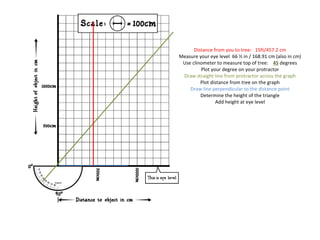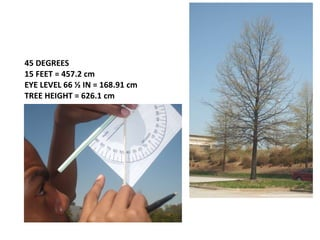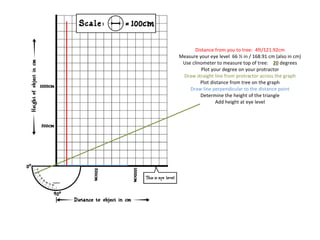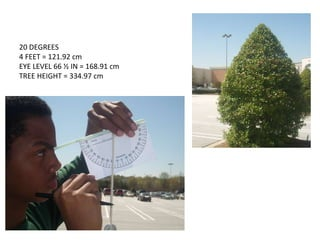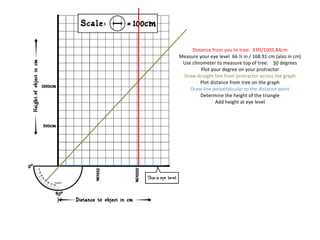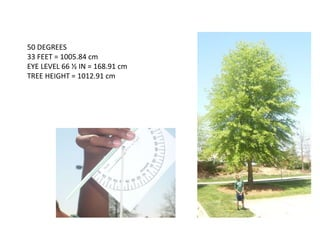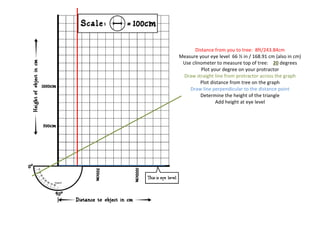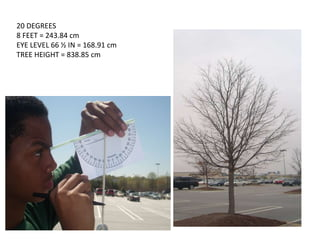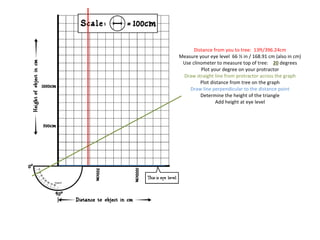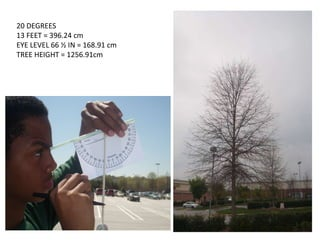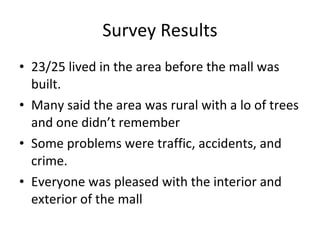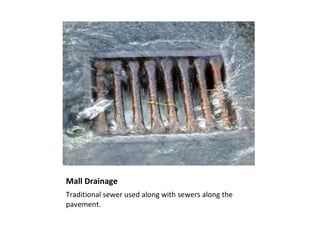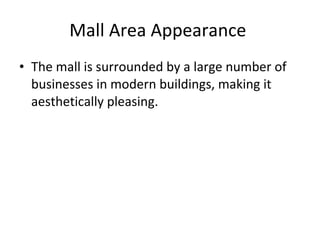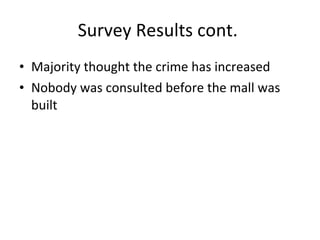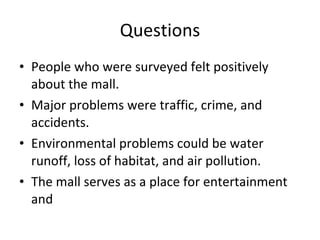1 of 19

### Apes mall project version 2 (1)

1. Land Use: Shopping Center Project By: Dorian Momon, Quantez Asbery, Aaron Williams, and Cameron Williams
2. Distance from you to tree: 15ft/457.2 cm Measure your eye level 66 ½ in / 168.91 cm (also in cm) Use clinometer to measure top of tree: 45 degrees Plot your degree on your protractor Draw straight line from protractor across the graph Plot distance from tree on the graph Draw line perpendicular to the distance point Determine the height of the triangle Add height at eye level
3. 45 DEGREES 15 FEET = 457.2 cm EYE LEVEL 66 ½ IN = 168.91 cm TREE HEIGHT = 626.1 cm
4. Distance from you to tree: 4ft/121.92cm Measure your eye level 66 ½ in / 168.91 cm (also in cm) Use clinometer to measure top of tree: 20 degrees Plot your degree on your protractor Draw straight line from protractor across the graph Plot distance from tree on the graph Draw line perpendicular to the distance point Determine the height of the triangle Add height at eye level
5. 20 DEGREES 4 FEET = 121.92 cm EYE LEVEL 66 ½ IN = 168.91 cm TREE HEIGHT = 334.97 cm
6. Distance from you to tree: 33ft/1005.84cm Measure your eye level 66 ½ in / 168.91 cm (also in cm) Use clinometer to measure top of tree: 50 degrees Plot your degree on your protractor Draw straight line from protractor across the graph Plot distance from tree on the graph Draw line perpendicular to the distance point Determine the height of the triangle Add height at eye level
7. 50 DEGREES 33 FEET = 1005.84 cm EYE LEVEL 66 ½ IN = 168.91 cm TREE HEIGHT = 1012.91 cm
8. Distance from you to tree: 8ft/243.84cm Measure your eye level 66 ½ in / 168.91 cm (also in cm) Use clinometer to measure top of tree: 20 degrees Plot your degree on your protractor Draw straight line from protractor across the graph Plot distance from tree on the graph Draw line perpendicular to the distance point Determine the height of the triangle Add height at eye level
9. 20 DEGREES 8 FEET = 243.84 cm EYE LEVEL 66 ½ IN = 168.91 cm TREE HEIGHT = 838.85 cm
10. Distance from you to tree: 13ft/396.24cm Measure your eye level 66 ½ in / 168.91 cm (also in cm) Use clinometer to measure top of tree: 20 degrees Plot your degree on your protractor Draw straight line from protractor across the graph Plot distance from tree on the graph Draw line perpendicular to the distance point Determine the height of the triangle Add height at eye level
11. 20 DEGREES 13 FEET = 396.24 cm EYE LEVEL 66 ½ IN = 168.91 cm TREE HEIGHT = 1256.91cm